Bare bones of neural networks

This is an intro to neural networks and does not assume prior knowledge. Theano is used here but almost identical code could be written for TensorFlow.

Neural networks are, like so many great ideas, in essence easy to understand but things get quickly out of hand if you increase the size or the complexity. That’s the reason why you have things like Keras, TFLearn and other meta-frameworks which hide the underlying plumbing of tensors and gradients.

The maths you need to grasp neural networks is fairly basic; some standard algebra and derivatives. If you look up the literature you encounter a bit more (at most path integrals) but it all boils down to very concrete and straighforward strategies. At some point things like quantum networks will become popular and engender a different kind of maths but for now things are easy.

First we’ll review the concepts and try to explain the basic ingredient; what is a tensor, what is a gradient and so on. Thereafter we tackle what a single neuron does or can do.

What is a tensor?

A tensor is simply a multi-dimensional matrix. From a strict mathematical perspective the word tensor is abused in the context of computer science. In reality a tensor is related to Riemannian manifolds and obeys precise transformation laws under coordinate transformations. So, it’s fine to use tensor as a synonym of multi-index matrix but don’t be too surprised if you look up in the literature what a tensor really is.

A tensor has an order or degree and a zero-tensor is also called scalar. It’s like a function or something returning a number. A 0-tensor on the real line is a simple real-valued function. Similarly, a 1-tensor is a vector and a 2-tensor is a 2d-matrix.

Tensors are used in neural networks because one transforms incoming vectors into different dimensions. Much like PCA in machine learning, things are sometimes crushed to lower dimensions. When dealing with images one linearized a 2d-object into a 1d-object. Or imagines that you have a digital marketing model with a latitude/longitude, browser caps and whatnot. If you bring it all together in one tensor you have many ways to observe visitors. It could also be the input for a neural network.

What is a neuron?

A neuron is a little box which receives some input and output some stuff. It can receive one number or many, it can output sound or images or anything you like. People usually describe a neuron as something which receives a vector $x$ and then transforms it like

$latex \sigma(w.x+b)$

with $latex w$ is a matrix called the weights, $latex b$ another vector called the bias and $latex \sigma$ a so-called activation function. Nothing prevents you to use something else. If you can find a way to use a neuron which predicts the arrival of a train on the basis of

$latex \theta(\sin(u.x)^7x)$

you will be rich and famous. That is, anything is valuable but certain things are easier to approach than others. The statement

$latex w.x+b$

is a linear transformation and linear things are easier than non-linear. Much of the successes of science are based on linearizations of complex phenomena. For example, elasticity is linear if you don’t stretch things too far. Describing an oscillator is based on a linear force. The thing is that coupling many linear things leads to complexity. If you couple many oscillators you get chaotic phenomena. Similarly, if you combine neurons which intrinsically have a linear mapping from a vector to another vector you can get complex output. Cellular automata are also a great example of how simple transformations lead to intensily complex output.

The activation function can be seen from different angles. It’s useful because it can switch on/off the output. It can also be seen as a way to increase the contrast in the linear transformation.

Let’s give an example. One of the often used activation functions is the sigmoid or smooth step-function. It’s like the step function but with a smooth transition. The smoothness matters also from a math perspective because this kinda function can be differentiated (technically; it’s continuous and differentiable).

import numpy as np
import theano.tensor as T
from theano import function

# the sigmoid
def sigma(x, alpha = 1): return 1/(1+np.exp(-alpha*x))

If you plot it with various values for alpha you will see that the higher the alpha value the more the step is strict/steep.

plt.plot(np.arange(-2,2,0.1), sigma(np.arange(-2,2,0.1), 5))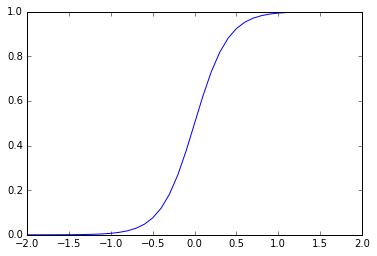Now, let’s take the linear transformation (with an arbitrary matrix w, here random) and see what the sigmoid does to the transform.

input = np.arange(-2,2,0.1)
w = np.random.randint(1,10,(40,40)) # whatever
b = np.random.randint(1,10,40) #whatever
# the normal linear transformation
linear_output = np.dot(w,np.arange(-2,2,0.1)) + b
# the sigmoid applied after the linear
smooth_output = sigma( np.dot(w,np.arange(-2,2,0.1)) + b, 5)
plt.figure(1)
plt.plot(input, linear_output)
plt.figure(2)
plt.plot(input, smooth_output)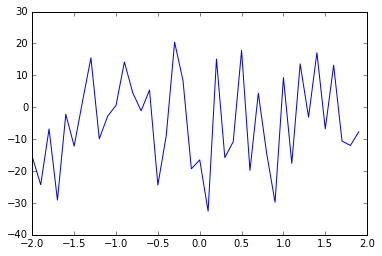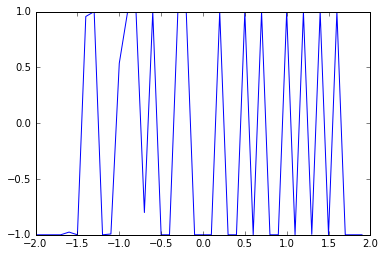The sigmoid stretches the output and is like increasing the contrast in an image. It dissipates the irregularities and increase the true signal. So, in a neural network this helps to reduce the propagation of noise or less important information as data moves across the network.

Another popular activation function is based on the hyperbolic tangent:

input = np.arange(-2,2,0.1)
w = np.random.randint(1,10,(40,40)) # whatever
b = np.random.randint(1,10,40) #whatever
# the normal linear transformation
linear_output = np.dot(w,np.arange(-2,2,0.1)) + b
# the sigmoid applied after the linear
smooth_output = np.tanh( np.dot(w,np.arange(-2,2,0.1)) + b)
plt.figure(1)
plt.plot(input, linear_output)
plt.figure(2)
plt.plot(input, smooth_output)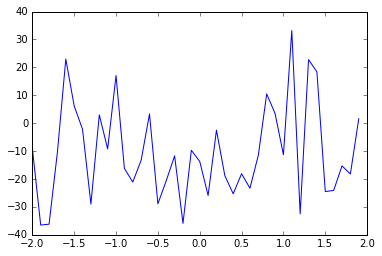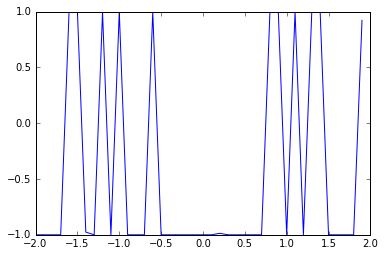As you can see, the tanh has a similar effect. At this point it’s useful to emphasize that there are no hard criteria to decide which activation function to use. Anything works and ultimately the accuracy of what you are trying to do justifies the choice of an activation function.

For example, if you take something like the sine function you emphasize the signal around the origin (with proper rescaling of the axis):

input = np.arange(-2,2,0.1)
w = np.random.randint(1,10,(40,40)) # whatever
b = np.random.randint(1,10,40) #whatever
# the normal linear transformation
linear_output = np.dot(w,np.arange(-2,2,0.1)) + b
# the sigmoid applied after the linear
smooth_output = np.sin((np.dot(w,np.arange(-2,2,0.1)) + b+2)*np.pi/4)
plt.figure(1)
plt.plot(input, linear_output)
plt.figure(2)
plt.plot(input, smooth_output)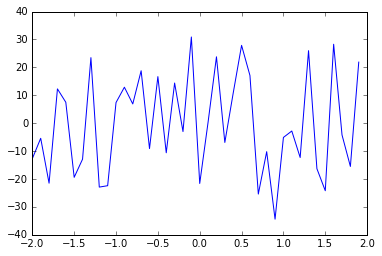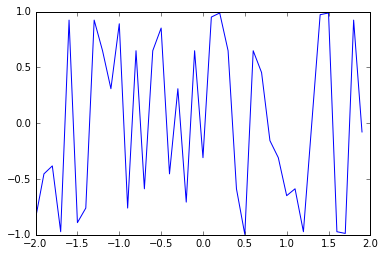So, there you go. A single neuron takes in some numbers and outputs some numbers. In practive one uses a linear transformation with an activation function on top of it.

Side note: why is linearity so useful?

The linear transformation $latex w.x+b$ can be seen as a transformation or a separation. If you ask when the transformation is zero, that is

$latex w.x+b==0$

you effectively define a separation in space. You divide the vector space in two regions. From a machine learning point of view this is equivalent to defining a classification.
Of course, real-world problems are never mathematically clean so separating a whole space with one line never works well. Let’s say the separation works well for negative numbers but is a different separation for positive numbers. In that case you need to define two linear transformations and switch from one to the other halfway. This ‘switching’ is precisely where activation functions come in: you keep the linear separation but you switch things on/of with the sigmoid (or whatever works best).
At the same time, many quirky separations (i.e. w iggling line) can be approximated by means of linear segments. This is in fact a common trick in maths: you defined something for segments and then generalize the whole lot by approximating the complex by means of linear segments. That’s how integrals are defined, that’s how simplices and differentiable manifolds are defined. So, here as well. If you have a complex machine learning problem you can approximate the complex separation by means of linear separations which act only within a small (sub)domain.

Practice makes perfect: optimization and gradients

Imagine darts or hunting or whatever shooting activity you prefer. You aim and the visual feedback tells you whether to shift a little to the right/left/whetever. You feed the arrow with a vector (the direction) and the difference between your aim and the place where the dart landed is an indication of how to adjust. Big mistake means big shift. The closer you are to the hotspot the finer the shift.

Training a neuron (or network in general) is very much the same. You want some output and compare the reality with what you wish to adjust the weights of the neuron.

Now remember that the bias is not a weight? There is an easy trick to make it appear as a weight. You extend the input with one dimension, assign 1 to the extra dimension and you are done. It’s a bit like looking at Euclidean spaces from a projective point of view; you add an extra dimension to deal with infinity. So, the transformation becomes homogenous and the weights and bias can all be treated in the same way when training a network.

The crucial things about adjusting things is; how does one know how to adjust the weights in order to increase the precision? That’s where gradients and derivatives come in.

Imagine you are skiing and you want to get down to the valley as quickly as possible. The slope is crucial here, the steeper the faster. Similarly, if you have a function which tells you how far you are away from your aim you take the tangent and follow that route to get as fast as possible to the minimum of the function. In the context or neural networks (by the way, the same happens when dealing with genetic algorithms) this is called the error function. The error function tells you the difference between the output and the value you actually would like to have. Obviously, the lower the error the better your network performs.

So, here is the great principle of the so-called back-propagation algorithm: you start with random weights, see what comes out, look at the error and adjust the weights according to the gradients/tangents of the error function to get as quickly as possible to the minimum of the error function.

It’s called back-propagation because you go back to your network to tell it how well it performed. Of course, much like going down to the valley while skiing, the terrain changes as you go and you cannot assume that the direction you take will remain constanst; you need to adapt to the terrain in small chunks. Similarly, you achieve perfection with a neural network by improving things in chunks. This is called epochs and refers to the fact that you need to feed the network many times with the available data to adjust the weights.

The mathematical translation of a slope or tangent is the concept of derivative of a function. This is not the place to explain calculus and there are plenty of resources on the net to see the light. It should be clear in any case that matrices, functions and derivatives are variable objects in a neural network. So, you should understand that a framework which deals with neural nets has to be capable to take the derivative of functions and deal with arbitrary tensors. Obviously, not all functions are meaningful (anyone seen incomplete Besself functions for activation?) and, hence, typical frameworks like TensorFlow and Theano deal with a limited set of functions.

Basic Theano mechanics

Plain and lengthy explanations aside, let’s see how neural networks are made possible in Theano. It should be clear at this point that there are some main ingredients when dealing with neural networks:

• tensors or matrices
• functions
• derivatives
• optimization (how to get somewhere as fast as possible)

So, let’s review how these things are done in Theano.

Things in Theano (and TensorFlow) are abstract and not defined directly like ordinary variables. There are two reasons for this:

• things defined in these frameworks run both on CPU and GPU, so you need a meta-model which gets translated to the architecture where it will effectively run
• you need to take the derivative of a function sometimes and allowing things for arbitrary (Python) function is not possible, so one has a safe sandbox rather than using the generic function definitions.

With T as the namespace for Theano tensors you define a scalar as

a = T.dscalar('a')
b = T.dscalar('b')

and define abstract functions using the ordinary operations

c = a*b

or the proper Theano operators

c = T.mul(a,b)

If you want to effectively use such an abstract function you can do so by defining a map with the abstract variables:

f = function([a,b],c)
f(2,3)
array(6.0)
You can define similarly abstract 1-tensors
v = T.dvector('v')
f = function([v], v**2)
f(np.repeat(2,3))
array([ 4.,  4.,  4.])

and so on with T.dmatrix, T.dtensor3 etc. As mentioned above, the framework has some differential calculus integrated. So, let’s define a more complex function

x,y,z = T.dscalars('x','y','z')
func = T.sinh(x**7 + T.exp(y*T.arccosh(z)))

# you should look up the names of the params, they can be useful to read the code. Here wrt = with respect to.
# func_dx = T.grad(func, wrt = x)

and the effective abstract form of the derivate can be obtained via the Theano pretty print (pp) function

from theano import pp
pp(dfx)
'(((fill(sinh(((x ** TensorConstant{7}) + exp((y * arccosh(z))))), TensorConstant{1.0}) * cosh(((x ** TensorConstant{7}) + exp((y * arccosh(z)))))) * TensorConstant{7}) * (x ** (TensorConstant{7} - TensorConstant{1})))'
Don’t worry about the whole expression, it’s an example demonstrating the fact that Theano can deal with some calculus. If you like this sort of stuff you should take a look at the Theano documentation which also highlights other calculus-related constructs like the Hessian and Jacobian. These things have to do with transformation of coordinates, integrals and more. In most cases you will not deal directly with these objects but if you implement your own neurons you will encounter them.

Now, how do we use all of this to optimize a neuron? Let’s take an even easier situation; finding the minimum of a function. The general problem is that of finding an extremum which includes both the minimu and the maximum. The mexican hat function is a good example because it exhibits both a maximum and multiple minima. This function is the prototypical example of how spontaneous symmetry breaking leads to mass (via the Higgs boson) in particle physics but that’s another story.

def mexican_hat_func(x, s=1): return (1-(x**2)/s**2)*np.exp(-x**2/s**2)
input = np.arange(-2,2,0.1)
plt.plot(input, mexican_hat_func(input))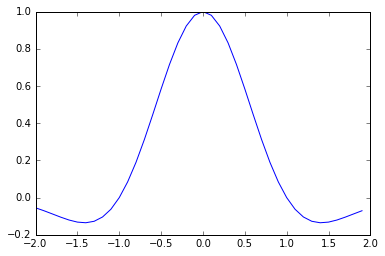Let’s try to find the right minimum by means of the slope. This is the so-called gradient descent approach. You start somewhere, follow the slope and try to reach the valley. In this case we use the variable w as the to-be-found weight to minimize the mapping of values around 1. That is, our x-values are

x = np.arange(0.9,1.1,0.01)
and we try to find a value for w such that the sum
def error(x, w=1): return (1-(w*x)**2)*np.exp(-(w*x)**2)
np.sum(error(x))

0.0845462594395677
is minimized. Think of the mexican hat as a neuron, the x-values as input and the aim to reach zero as output.
input = np.arange(0.9,1.1,0.01)
markers_on = mexican_hat_func(input)
plt.plot(np.arange(-2,2,0.1), mexican_hat_func(np.arange(-2,2,0.1)))
plt.plot(input, markers_on, marker='x', color='red')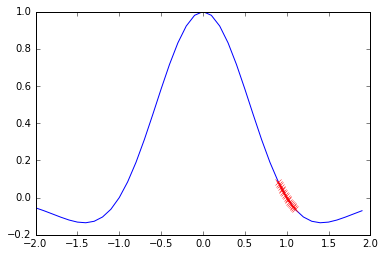If take the derivative of the hat and set this to zero (the usual way to find an extremum) you will see that the minima are at +/- the square-root of 2. Using the Theano mechanics we can see this by defining first some functions

input = np.arange(0.9,1.1,0.01)
x = T.dscalar('x')
w = T.dscalar('w')
func = (1-(w*x)**2)*np.exp(-(w*x)**2)
error = function([x,w], func)
# sum the mapped values
def total_error(w):
total = 0
for i in range(len(input)):
total += error(input[i], w)

print(total_error(0.5))
12.2604829313
note that w is here a shared variable and not a scalar. The optimization with gradient descent is obtained by shifting systematically in the direction of the gradient:
epsilon = 0.01
weight = 0.5
shift = function([x,w], dw)
def shift_weight(weight):
avg = 0
for i in range(len(input)): avg += shift(input[i], weight)
avg = avg/len(input)
#negative derivate means pushing to the right
weight -= epsilon*avg
return weight

r = []
for i in range(400):
weight = shift_weight(weight)
r.append(total_error(weight))
plt.plot(r)
print("The optimized value of the weight is: %s"%weight)

The optimized value of the weight is: 1.41592163386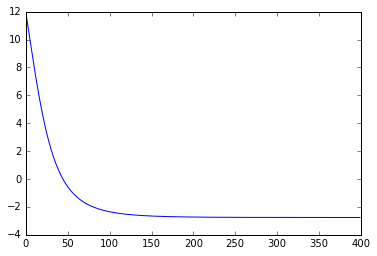The reason why the convergence does not go to zero (as you might expect) is because the precise minimum is the sum with the exact square root of two:

np.sum(mexican_hat_func(input*np.sqrt(2)))
-2.7585434222761691
which corresponds with what you see in the line-plot above.
Of course all of this is rather tedious and is only to show you how the whole optimization of a neuron works. Theano has a much more direct way to reproduce this:
input = np.arange(0.9,1.1,0.01)
x = T.dvector('x')
w = theano.shared(0.5)
func = T.sum((1-(w*x)**2)*np.exp(-(w*x)**2))
learning_rate = theano.shared(0.001)
train = function(
[x],
outputs = [w,func],
(w, w-learning_rate*dw)
]
)
r = []
for i in range(300):
r.append(train(input))
plt.plot(r)
print("The optimized value of the weight is: %s"%r.pop())
The optimized value of the weight is: 1.4215863505344246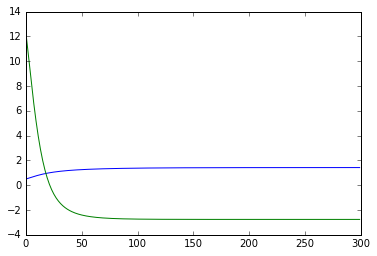At this point it’s probably good to add a few remarks:

• the example above can be generalized in all directions; more neurons, different tensorial dimensions, different optimizations and more
• frameworks like Theano an TensorFlow enable the construction of arbitrarily complex topologies of coupled neurons and run on top of both cpu and gpu
• you don’t need to fully understand all the intricacies of Theano to use it. If a new research paper demonstrates a faster way to get to a minimum value it likely gets integrated in, say, TensorFlow but the higher level functions remain the same
• the backpropagation works with this simple type of neuron (aka perceptron) but one needs more mechanics to deal with so-called recurrent networks and other topologies. A neural network considered as a graph can have loops and create situations where data keeps flowing or does not converge
• convergence of weights is a topic on its own. There are theorems proving the convergence of weights and bias based on certain conditions but there is in general no guarantee that training a network leads to converging parameters
• our mexican hat has actually two minima and one can have in general many extrema and minima which are not global minimums. This is, as well, a whole research topic.
• neural networks are like flows of tensors (hence the appropriate name TensorFlow) but real-world data often needs to be preprocessed before one can feed a net. Sentences, images and sound can be converted to tensors in various ways and while there are standard approaches there is no mathematical or other constraint to approach things differently. The whole field of neural networks is a much an art and a craft as it is a science. To some extend, if something works for your situation, that’s OK. Even if there isn’t any literature proving it should work.
• training a network is a costly thing. The convergence above is obtained after 300 loops. In general, one has to use highly parallelized algorithms on GPU’s to obtain things in a timely fashion. Microsoft and other giants typically run experiments on hundreds of GPU’s sometimes in a span of weeks. So, if things seem slow on your end, don’t imagine the magic of neural networks comes cheap
• Theano does not come with high-tech optimization algorithms but TensorFlow does. Theano does not have for example the so-called adadelta optimizer (among other). Both frameworks have pro/con and all depends on what you want to do. Meta frameworks like Keras and TFLearn have even more hidden powers but you are less in control
• the training of a network does not necessarily have a unique solution. Some networks can have infinitely many solutions. Some have none. To prove or disprove things mathematically is quite difficult. Usually you need to simulate things and see what gives. Experience and intuition are your friends.

Some examples

Now that the general concepts and the Theano mechanics is explained, let’s see what one can do with neurons.

Boolean logic

Basic neurons can simulate boolean logic. Take the AND operator, it’s straightforward to implement it.

from theano.ifelse import ifelse

x = T.vector('x')
w = T.vector('w')
b = T.scalar('b')

z = T.dot(x,w)+b
a = ifelse(T.lt(z,0),0,1)

neuron = theano.function([x,w,b],a)

We’ll train the neuron in a moment but let’s first show that there is a solution:

inputs = [
[0, 0],
[0, 1],
[1, 0],
[1, 1]
]
weights = [ 1, 1]
bias = -1.5

for i in range(len(inputs)):
t = inputs[i]
out = neuron(t,weights,bias)
print("%d AND %d = %d"%(t,t,out))
0 AND 0 = 0
0 AND 1 = 0
1 AND 0 = 0
1 AND 1 = 1

Cool, it works with this solution. Can we discover it?

from random import random

x = T.matrix('x')
w = theano.shared(np.array([random(),random()]))
b = theano.shared(1.0)
learning_rate = 0.01

z = T.dot(x,w)+b
a = 1/(1+T.exp(-z))

a_hat = T.vector('a_hat')
cost = -(a_hat*T.log(a) + (1-a_hat)*T.log(1-a)).sum()

train = function(
inputs = [x,a_hat],
outputs = [a,cost],
[w, w-learning_rate*dw],
[b, b-learning_rate*db]
]
)

#Define inputs and weights
inputs = [
[0, 0],
[0, 1],
[1, 0],
[1, 1]
]
outputs = [0,0,0,1]

cost = []
for iteration in range(30000):
pred, cost_iter = train(inputs, outputs)
cost.append(cost_iter)

print( 'The final/optimized output is:')
for i in range(len(inputs)):
print('%d AND %d is %.2f' % (inputs[i],inputs[i],pred[i]))

import matplotlib.pyplot as plt
%matplotlib inline
plt.plot(cost)
print("\nThe optimized weights are:")
print("w: %s"%w.get_value())
print("b: %s"%b.get_value())

The final/optimized output is:
0 AND 0 is 0.00
0 AND 1 is 0.02
1 AND 0 is 0.02
1 AND 1 is 0.98

The optimized weights are:
w: [ 7.78215595  7.78215584]
b: -11.845091775640519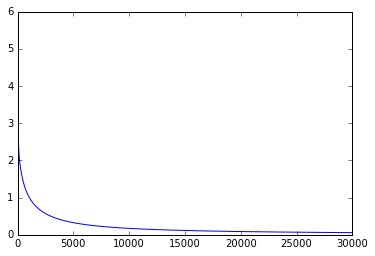The result of this training shows that we get a completely different but equally functional estimate of the weights. This demonstrates the non-uniqueness of neural network weight distribution.

If you wonder where the odd error or loss function comes from, this is called the cross-entropy loss function and is fairly standard way to compare results and targets which supposedly are binary. It has its origins in information theory.

Side note: using R for an AND network

The package neuralnet in R allows you to create an AND gate with much less code:

library(neuralnet)
AND <- c(rep(0,3),1)
binary.data <- data.frame(expand.grid(c(0,1), c(0,1)), AND)
print(net <- neuralnet(AND~Var1+Var2, binary.data, hidden=0, rep=10, err.fct="ce", linear.output=FALSE))
plot(net, rep="best")
prediction(net)

The great thing about this R package is that is also allows you to plot the network.Maybe something which is also not immediately clear from the whole discussion above is that you can create networks which simulates multiple functions at the same time. Say, you want to output both AND and OR with the same net:

AND <- c(rep(0,7),1)
OR <- c(0,rep(1,7))
binary.data <- data.frame(expand.grid(c(0,1), c(0,1), c(0,1)), AND, OR)
net <- neuralnet(AND+OR~Var1+Var2+Var3,  binary.data, hidden=0, rep=10, err.fct="ce", linear.output=FALSE)
plot(net, rep="best")
prediction(net)

Have fun, you can create in principle any real-value function in this fashion. Yes, literally, there are hard mathematical proofs of this fact and it’s known as the universal approximation theorem or the Hornik theorem.

You can easily play with this fact in R, here an example of approximating the sine function with a deep network:

d = data.frame("x" = 1:20, "y"= sin((1:20)^2))
fit <-neuralnet ( y~x ,
data=d ,
hidden =c(3 ,3), linear.output=FALSE, likelihood=TRUE)
plot(fit, rep="best")
p=prediction(fit)

plot(d, t="l", col="green")
par(new=T)
plot(p\$data, col="red", t="o")

What does it take to compute an AND and OR gate at the same time in Theano. You simply define two neurons and optimize them in the same loop:

x = T.matrix('x')
w1 = theano.shared(np.array([random(),random()]))
b1 = theano.shared(1.0)
w2 = theano.shared(np.array([random(),random()]))
b2 = theano.shared(1.0)
learning_rate = 0.01

# the standard sigmoid neuron
and_neuron = 1/(1+T.exp(-T.dot(x,w1)-b1))
or_neuron = 1/(1+T.exp(-T.dot(x,w2)-b2))
# the aim or target vector
t1 = T.vector('t1')
t2 = T.vector('t2')
# cross-entropy evaluation
cost = -(t1*T.log(and_neuron) + (1-t1)*T.log(1-and_neuron)).sum() -(t2*T.log(or_neuron) + (1-t2)*T.log(1-or_neuron)).sum()

train = function(
inputs = [x,t1,t2],
outputs = [and_neuron, or_neuron, cost],
[w1, w1-learning_rate*dw1],
[b1, b1-learning_rate*db1],
[w2, w2-learning_rate*dw2],
[b2, b2-learning_rate*db2]
]
)

#Define inputs and weights
inputs = [
[0, 0],
[0, 1],
[1, 0],
[1, 1]
]
target1 = [0,0,0,1]
target2 = [0,1,1,1]

cost = []
for iteration in range(30000):
and_pred, or_pred, cost_iter = train(inputs, target1, target2)
cost.append(cost_iter)

print( 'The final/optimized output is:')
for i in range(len(inputs)):
print('%d AND %d is %.2f' % (inputs[i],inputs[i],and_pred[i]))
print('%d OR %d is %.2f' % (inputs[i],inputs[i],or_pred[i]))
The final/optimized output is:
0 AND 0 is 0.00
0 OR 0 is 0.02
0 AND 1 is 0.02
0 OR 1 is 0.99
1 AND 0 is 0.02
1 OR 0 is 0.99
1 AND 1 is 0.98
1 OR 1 is 1.00

At this point it should become clear that devloping more complex neural topologies leads to complex expressions and series of variable definitions. This is were Theano stops being useful and where meta-frameworks step on stage. Theano should be considered like simpy or some other low-level abstract computation framework. If you want to create some real-world neural networks you need to move beyond Theano.

In another article we’ll explain how Keras allows you to create more complex neural networks and how various topologies (e.g. recurrent neurons) can solve real-world problems.